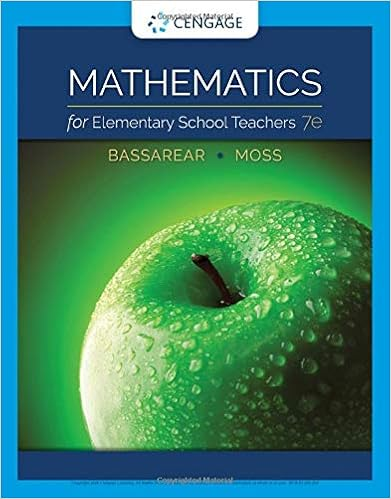# written homework 1 solutions.pdf - Kirstin Richards Written...

• Homework Help
• beckster744
• 1
• 100% (1) 1 out of 1 people found this document helpful

This preview shows page 1 out of 1 page.

##### We have textbook solutions for you!
The document you are viewing contains questions related to this textbook.The document you are viewing contains questions related to this textbook.
Chapter 4 / Exercise 37
Mathematics for Elementary School Teachers
BassarearExpert VerifiedUnformatted text preview: Kirstin Richards – Written Homework Week #1 1. ( → ) → ( → ) ≡ ¬( → ) ∨ ( → ) rule of implication ≡ ¬(¬ ∨ ) ∨ (¬ ∨ ) rule of implication ≡ (¬¬ ∧ ¬) ∨ (¬ ∨ ) 2nd De Morgan’s law ≡ ( ∧ ¬) ∨ (¬ ∨ ) Double negation law ≡ [( ∧ ¬) ∨ (¬)] ∨ associative law ≡ ¬ ∨ absorption ≡ → implication 2. “If xy is negative, then x is non-positive or y is negative.” < 0 → ≤ 0 ∨ < 0 3. Standard if then form a. “If we fly business class, then I will go on vacation to Australia with you.” b. “If your pets are healthy, then you care for them well.” c. “If you don’t make me a better offer, then I will keep my current job.” d. “If we observe faster than light travel, then we have a reason to question relativity theory.” 4. 3 ≤ < 4 means 3 is less than or equal to x and x is less than 4, or (3≤ ) ∧ ( < 4) ′ ℎ (3 ≤ ) ∧ ( < 4) ¬(3 ≤ ) ∨ ¬( < 4). ℎ ℎ (3 > ) ∨ ( ≥ 4) 5. Demonstrate that ( → ) ∧ ( → ¬) is not a contradiction a. The truth values that make the statement true would be FFTT. We know this because for this statement to be a contradiction all the truth values would have to be F. For all the values to be F. (p→q) has a truth table of TFTT. Whenever we encounter an conditional with only 2 variables we will only have one F in the set of 4 answers. This allows us to know that ( → ¬) will only have 1 F answer as well. When we incorporate the conjunction, we know that Anytime both equations are T the statement will be true and since each set of truth values only has 1 F answer the max number of F in the final truth values will only be 2 of 4 meaning that the solution could not be a contradiction because it will always have at least 2 T values. b. ( → ) ∧ ( → ¬) ≡ (¬ ∨ ) ∧ (¬ ∨ ¬) = ¬ ∨ ( ∧ ¬) ≡ ¬ ∨ ≡ ¬ ...
View Full Document

•••